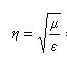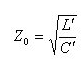## Difference between intrinsic and characteristic impedance

• 0
We got a question from Lauren, who asked me to post it on her behalf. Let's see if we can help her out! She wrote:

I am a young engineer from New Hampshire. I was wondering whethercould be used to define the characteristic impedance of a transmission line instead ofand if NOT, why? What is the fundamental difference between a "uniform material" and a transmission line? A transmission line also has a permittivity and a permeability... why can't we use them to calculate impedance? Another point of confusion: intrinsic and characteristic impedance seem to be used analogously for propagating EM signals in a uniform medium and on a TL, respectively, so why would they be defined differently?

If eta CAN be used in place of Zo, this seems to imply that in a transmission line, the ratio of permeability to permittivity is the same as the ratio between the per-meter inductance to capacitance. Why?

And tangentially, how do we measure / design for per-meter inductance and capacitance in real life (e.g., how do we ensure that our 50 ohm TL is as close to 50 ohms as possible)?

Thank you so much, and thank you for your wonderful website!
• 0
Dear Lauren,

Your first equation works for a plane wave. In other transmission lines, you need a version of the second equation. This is actually a complex question. I would start with "A general waveguide circuit theory" by Roger Marks and myself.

Dylan
• 0
Hi

For a plane wave propagating in a source free, lossless dielectric medium, the wave impedance formula sqr[mu/eps] describes the ratio of E to H fields.

In an ideal transmission line (TEM mode assumed here), the E and H fields propagate perpendicular to each other, and so the WAVE IMPEDANCE (The ratio E/H at any given point in space within the TL), is also defined by sqr[mu/eps].

In TL theory we work with "voltage and current", defining their ratio as the "Characteristic impedance of the transmission line": Zc=V/I.

But "V" and "I" are DERIVED QUANTITIES, derived from the fundamental E and H fields by means of line integrals of those fields in the transversal section of the TL, plus boundary conditions, like Vo and Io as applied currents or voltages. (Check the excellent paper by Dr. Williams or other references like Ramo, Pozar, Harrington etc.)

Thus "V" and "I" depend on the geometry and materials conforming the transmission line, and their ratio may or may not equal the quotient E/H. Thus the TEM wave impedance is equivalente to the plane wave impedance for a similar medium, but wave impedance and TL's characteristic impedance can differ.

And this is only for the TEM mode... If you start to consider TE, TM or hybrid modes, more and more "impedances" show up. And there are many situations (e.g.:In a hollow rectangular waveguide) where the derivation of V and I themselves as integrals of E and H fields is not straitforward, or even unique.

Regarding the microstrip, the fundamental mode is not a pure TEM but a quasy-TEM mode: Permitivities differ in substrate and air, and thus there are longitudinal E and H fields to maintain continuity. Nonetheless, for lower frequencies this longitudinal components can be neglected and the static solution is taken as the spatial distribution of E and H. You can compute from this field distribution the C and L values of your transmission line.(e.g.: Pozar, 3.8)

Hope this helps.

Iban
• 0
Characteristic impedance does not even need a transmission line, there is a characteristic impedance associated with wave propagation in any uniform medium. The intrinsic impedance is a measure of the ratio of the electric field to the magnetic field.
bold
italic
underline
strike
code
quote
ulist
image
url
mention
reveal